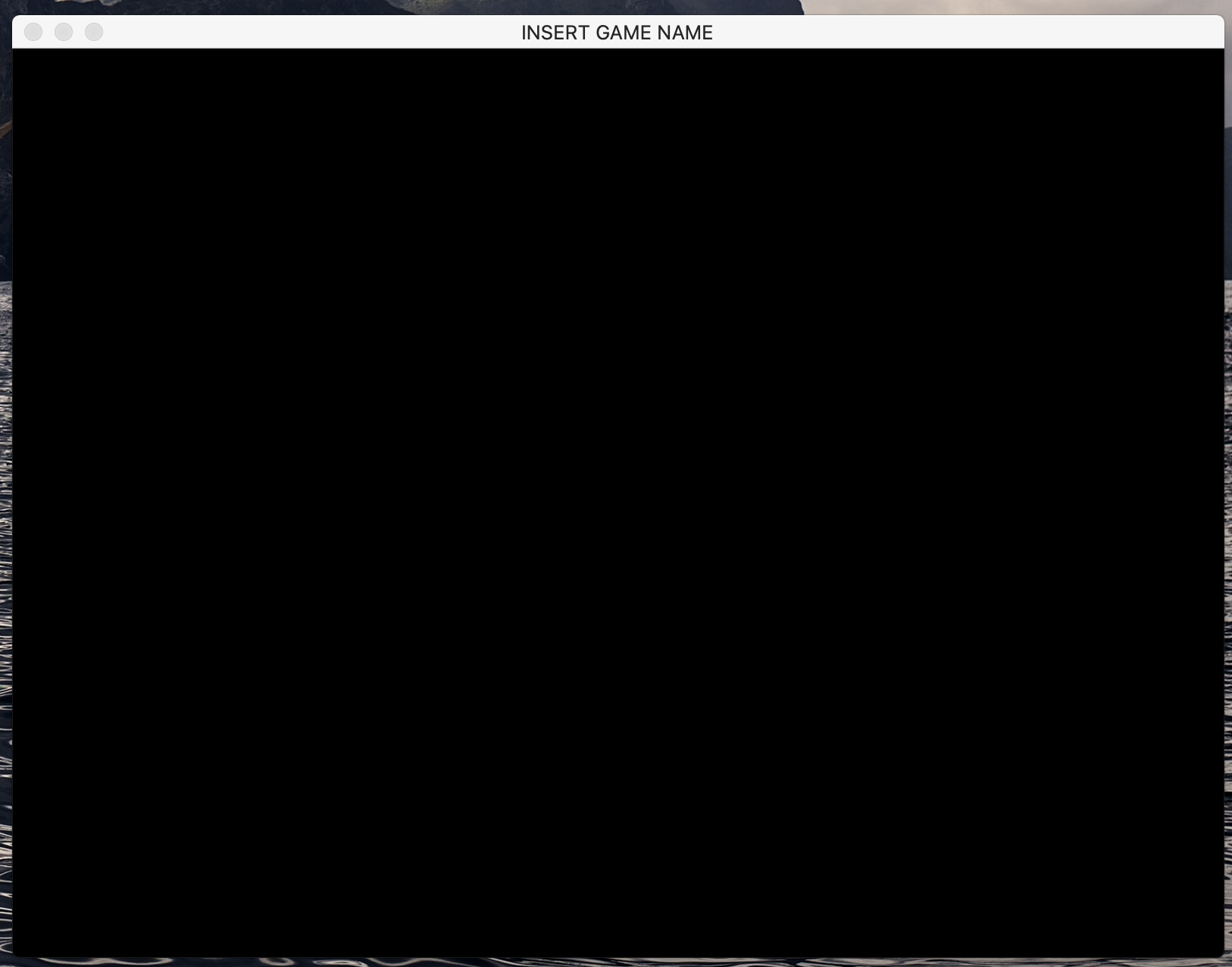Python
Share
Explore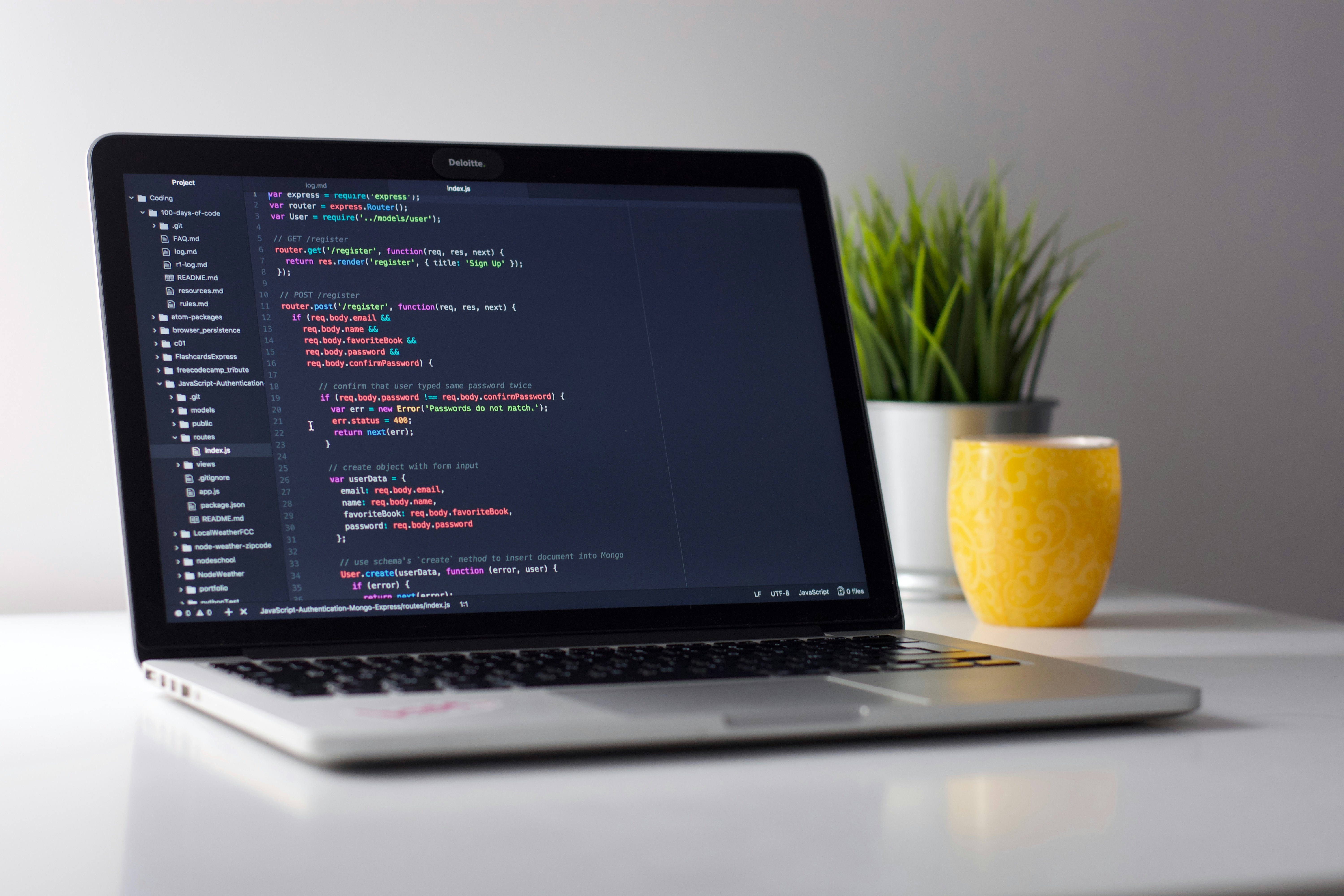Printing
The print function in Python is used very often to output something.
print(“name”)
Output -> name
What Integers, Variables, Lists, Strings are

Integers -> Numbers
Strings -> Words with “”
Variables -> A place where you can store data
List -> A type of data that allows you to store multiple things
For example:
Integer: 2
String: “Hello”
Variable: name = “Sally”
List: list1 = [1, 3, 5, 2, 4]
How would you print Integers, Variables, Lists, and Strings?
Integers: print(______[write in integer, no “”])
Variables: print(_______[write in variable, no “”])
Strings: print(“______”[You can write in anything you want as long as it’s in quotes])
Lists: print(_______[Write the variable to store in the list]])
Examples:
Printing Integers:
print(2)
Printing Varibles:
name = "Sally"

print(name)
Printing Strings:
print("Hello")
Printing Lists:
List1 = ["hi", "this", "is", "a", "list"]

print(List1)
Input()

What is input?
→ Input is when the user gets to choose

For example:
print(“Which color do you like best? Pink or blue.”)
Color = input()
Name = input()
print(“Hello, my name is ” + Name + “. And I like the color ” + Color + “!”)
Output:
→ Hello my name is Sumay. And I like the color blue!
As you can see input() is really cool! Let’s see if we can expand on it!
Indexes

Food = [“Apple”, “Banana”, “Peach”, “Watermelon”, “Kiwi”]
^ ^ ^ ^ ^
0 & -5 1 & -4 2 & -3 3 & -2 4 & -1
Indexes: They point to a specific element in a list
Positives:
print(Food) —> Apple
print(Food) —> Banana
print(Food) —> Peach
print(Food) —> Watermelon
print(Food) —> Kiwi

Negatives:
print(Food[-5]) —> Apple
print(Food[-4]) —> Banana
print(Food[-3]) —> Peach
print(Food[-2]) —> Watermelon
print(Food[-1]) —> Kiwi

Printing with +
Sometimes you want to print multiple things in one print statement. This is made possible by using a + sign in your print statements!
Word = "Carrots"
print("My favorite food is " + Word + ".")
Output → My favorite food is Carrots.
--
Numbers = [1,2,3]
Numbers2 = [4,5,6]
print(Numbers + Numbers2)
Output->[1,2,3,4,5,6]
all_numbers = (Numbers + Numbers2)
print(“These are some of the numbers I know! ” + all_numbers)
Output->These are some of the numbers I know! [1,2,3,4,5,6]
len()

len() it counts how many items are in a list.
Number = [1,2,3,4,5,6,”Hello”]
print(len(Number))
Output —> 7
print(len(Food))
Output -> 3

Append

Monkey = [“M”, ”o”, ”n”, ”k”, ”e”]
Monkey.append(“y”)
That adds to the list so it has the word “monkey”.
print(Monkey)
Output —> ['M', 'o', 'n', 'k', 'e', 'y']

Del()

Del() is used to delete things from a list.
Syntax:
del(list name[index])
x = [1,2,3,4,6,”hello”]
print(x)
Output —> [1,2,3,4,6,’hello’]
I wanna delete 6
del(x)
print(x)
Output —> [1,2,3,4,’hello’]
len(x)
Output—> 5
If and Else Statements

Box = “toy”
If Box == “toy”:
Box = empty
Else:
Box = toy

Why is the difference between = and ==?
= means assign == means compare.

Elif

Elif is for when there is another if
For example:
apple = input()
If apple == “red”:
Elif apple == “green”:
Else:

Casting
Casting is converting integers into string and vice-versa

For example:
How would you change 3 into a string.
str(3)-> “3”
Syntax:
str(*integer*)
Or a string into an integer.
int(“3”)-> 3
Syntax:
int(*string*)

Functions

What are functions?
Functions are quick ways of running big amounts of code.

Syntax:
def *Function name*():
*What you want the function to do*
*Function name*()
print(“Hi”)
print(“My”)
print(“Name”)
print(“Is”)
print(“Bob”)
Output—> Hi
My
Name
Is
Bob
We can make a function:
def Printing():
print(“Hi”)
print(“My”)
print(“Name”)
print(“Is”)
print(“Bob”)
return Printing

Printing()
Output—> Hi
My
Name
Is
Bob
The thing about a function is that you can do it multiple times!
Printing()
Printing()
Output—> Hi
My
Name
Is
Bob
Hi
My
Name
Is
Bob
You can also have customizable functions where you have to input values in the () in your function.
def persontemplate(firstname, lastname, age):
print("First Name: " + firstname)
print("Last Name: " + lastname)
print("Age: " + age)

persontemplate("Jeff", "PersonDude", "155")
persontemplate("Sally", "Hello", "68")
By adding (firstname, lastname, age) in the function you make it so that when you call the function, you have to add those values.

How to make things uppercase

String1 = “Hello”
print(String1.upper())
Output → HELLO
print(String1.lower())
Output → hello
13. How to make things lowercase
String1 = “HELLO”
print(String1.lower())
Output → hello
For loops

A For loop loops through a list.
x = [1,2,3,4,5]
If you want to change something in a list you can do this:
x = 2
print(x)
Output—> [2,2,3,4,5]
What if you want to change x into:
[3, 3, 4, 5, 6]
That would take a long time.
So we can make a For loop.
This for loop would add 1 to every element of the list.
Syntax:
For *variable to hold the current element of the list* in *list*():
___________
___________
Then it does whatever you put for the for loop to do and does it to every element in the list.
For i in x():
i = i + 1
How to keep track of points

How would you keep track of points in python?
→ Unfortunately there is no built in function that keeps track of points. So we have to build it ourselves.
Let’s get started!
# First we make a variable called points.
Points = 0
# We start with 0 because we start out with no points
# Now, we need to have something to put points for.
# So, we are going to make a quiz!
# Let me show you an example so you understand.
print(“What is my favorite color?”)
# My favorite color is black.
Points + 1
print(“Correct!”)
Else:
Run:
What is my favorite color?
Correct!
# Now you make an example!
# After it works, then we can make our quiz longer!

# There are three important things that will be used a lot in python
# Lets start with learning what they are and how to use them
# These three things are integers, variables, and strings
# What are Integers?
"""
Integers are numbers.
For example: 1 or 5
How do print Integers?
Well, lets first start out by saying what printing means
When you print something, you are just showing people data or information
This information can also include integers, or as we just learned, numbers
How you print something is pretty simple...
print()
That's how you do it
The data that you want to show will go inside these things --> ()
When printing an integer you don't have to anything except put the integer inside --> ()
So, if I wanted to print the integer 3, I would do this
print(3)
Can you print three different integers for me?
"""

"""
Next, what is a variable?
A variable is a place where you store information
After you store the information in a variable you can also print the information inside the variable
An example of a variable is this:
number = 4
number is basically the name of the variable
Inside of number, we put in information
This information is an integer
To print the integer inside the variable we do this
print(number)
We do kind of the same thing, but instead of putting in an integer, we put in a variable
Since the variable has an integer inside it, python will print the integer
If we accidentally put in the wrong name for the variable when we are trying to print it python will give us an
error
And error is when something goes wrong in the code that we write and python can't do what we want it to do
So if I did this by accident
print(nombar)
Python will give me an error and will say it was not able to print the integer
Can you make three variables for me?
"""
# Make the variables here

"""
Now can you print the variables?
"""
# Print the variables here

"""
"""
While Loops
*In progress*
Turtle
Turtle is a really cool package you can import into your python project to make graphics and drawings with your code. The first thing you need to do is at the top of your code write import turtle .
Before making the turtle do or draw anything, your have to write turtle. This might not seem like much but if you are going to write a lot of code you may want to make it shorter. You can do this by writing
ttl = turtle.Turtle() you can make the ttl anything you want. For example: bob = turtle.Turtle()
Making the turtle move and draw
To make the turtle draw the first thing you have to do is ttl.pendown(). Then when you make the turtle move it will drag a line behind it.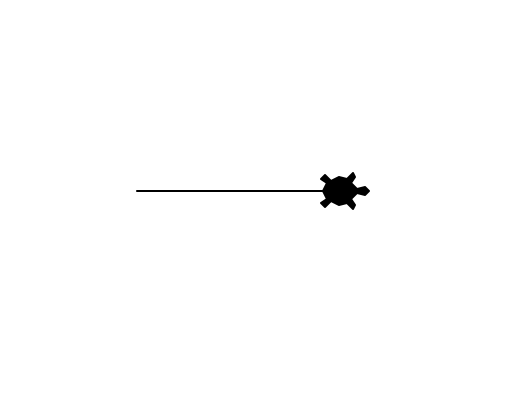The way you can make the turtle move is with four functions:
ttl.forward(100) # the (100) makes the turtle go forward 100 pixels
ttl.left(90) # the (90) makes the turtle turn 90 degrees to the left
ttl.backward(100) # the (100) makes the turtle go backward 100 pixels
ttl.right(90) # the (90) makes the turtle turn 90 degrees to the right
You can also make a circle by writing:
ttl.circle(50) # the (50) affects how pixels long the circle's radius is

Functions:
.pencolor - You are able to change the pen color that your turtle draws with by saying:
turtle.pencolor("blue")
Keep in mind that you need to make sure that the package turtle has the color you are using. Here is a chart with all the colors you can use: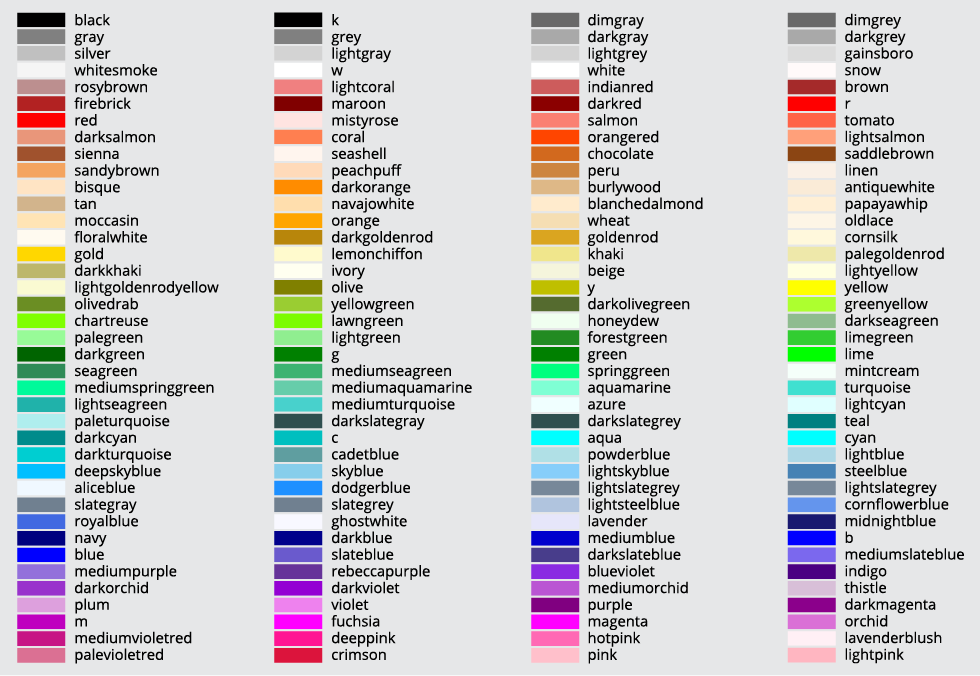.width - This allows you to change the with that you draw in by writing:
turtle.width("8")

.shape - This function allows you to change the shape of the turtle that is drawing the lines:
turtle.shape("turtle")
turtle.shape("arrow")
turtle.shape("circle")
turtle.shape("triangle")
turtle.shape("classic")

Cryptography
Cryptography is a way to encode and decode messages with python. This is useful for when you are sending sensitive information in a way that is safe from hackers. Here are some ways you can encode messages you are sending:
Reversing the message:
message = input()

def encode(message):
return ''.join(reversed(message))
If you inputted message as hi, then the output would be “ih”
The way this works is the “”.join bit makes it so that in between each character there is a “”, so nothing is put there, and the the (reversed(message)) part reverses the message. You can also put something in the “” to make it so that there is something placed between each character!
message = input()

def encode(message):
return '/'.join(reversed(message))
This would make it so that if message = “hello” the output would be: o/l/l/e/h
Which is a lot less readable.

Changing Position In Alphabet
The next way you can crypt a string is by changing the position in the alphabet. For example you could make it so that if you put in a letter, it will move 2 letters in the alphabet. So if you put in “a” the output would be “c”. Here’s the code for you to do it!

message = input("message: ").lower() # This allows you to put in the message
# The reason why I added .lower() is because when a letter is uppercase the encryption
# doesn't work

alphabet = "abcdefghijklmnopqrstuvwxyz" # This is so we can find the letter's position in
# the alphabet and then change it

encrypt = "" # This is an empty variable that we can use to store the encrypted message

for i in message: # This is a for loop that loops through every letter in the message
position = alphabet.find(i) # This finds the letters position in the alphabet and
# then stores in the variable position

newposition = (position+5) %26 # This variable changes the letter so it is five
# letters forward in the alphabet and then stores the new letter in newposition
# the %26 makes it so that if the message is "z" then to encrypt it, it will start

encrypt += alphabet[newposition] # Then it takes the new letter and then stores it
# in the encrypt variable. The for loop will make it so that every letter will be
# stored

print(encrypt) # This prints the encrypted message

question = input("Would you like to decrypt? (Yes/No)")
if question == "Yes": # If statement that checks to see whether you inputted Yes
decrypt = "" # Variable that will store the decrypted message
# This is just another for loop that will do the same thing as the other one except
# it will decrypt the message instead by doing "- 5" instead of "+ 5"
for i in encrypt:
position = alphabet.find(i)
newposition = position - 5
decrypt += alphabet[newposition]

print(decrypt) # Prints decrypted message
else: # Else statement if you didn't type Yes
print("Okay!")

MatPlotLib.Pyplot
Matplotlib is an extension to python that allows you to create charts and map data! The first thing you have to do is download the extension by running in your terminal (not the Python Shell):
python3 -m pip install matplotlib
Then you should import it at the top of your code like this:
import matplotlib.pyplot as plt
By saying “as plt” this makes it so that you can now use the package by calling plt instead of having to write out matplotlib.pyplot. Then you can use plt.plot([]) to insert what the graph should chart by using a list of numbers. You can also use the functions plt.ylabel(’’) and plt.xlabel(’’) to label the axis and what they represent.
After you have created what should be graphed and displayed, end your code with plt.show()
Example:
import matplotlib.pyplot as plt

plt.plot([1, 2, 3, 4])
plt.ylabel('Y Axis')
plt.xlabel('X Axis')

plt.show()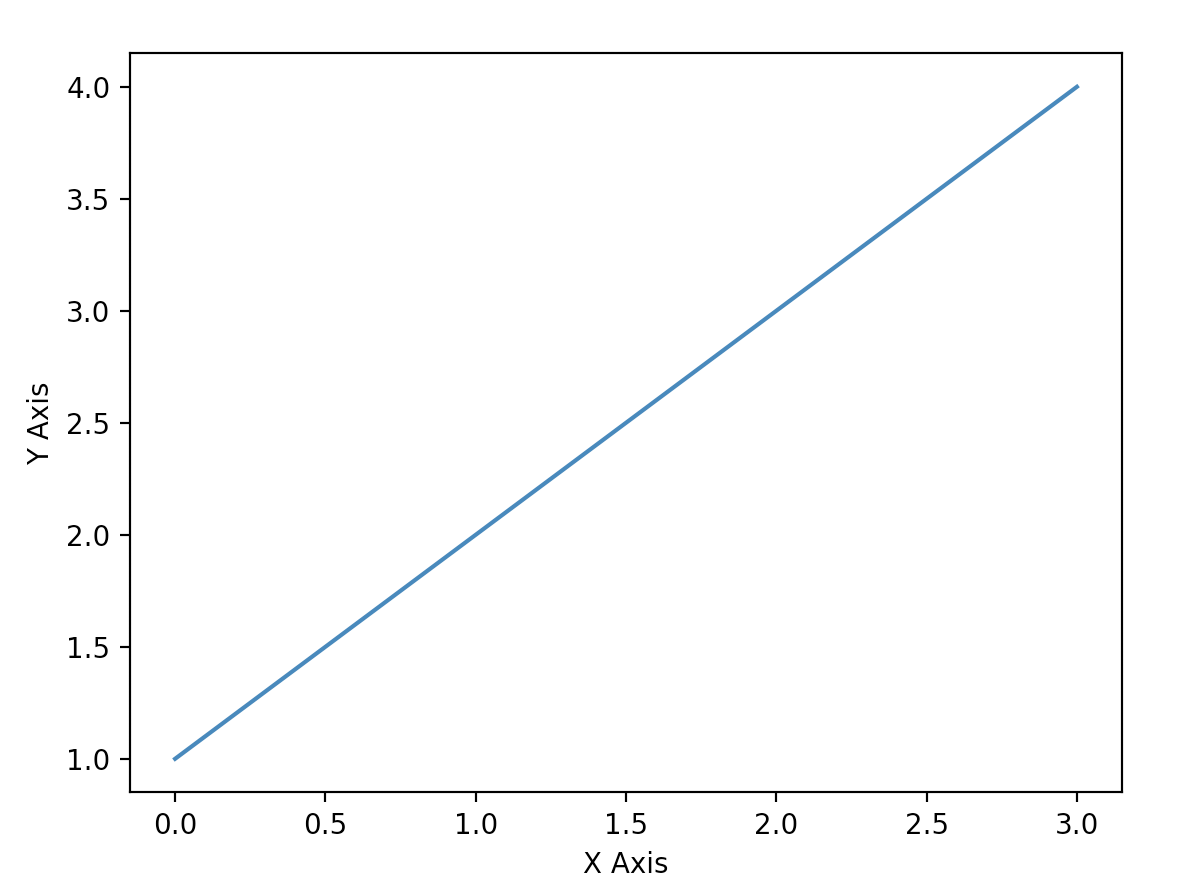To plot multiple lines, just call plt.plot() again:
import matplotlib.pyplot as plt

plt.plot([1, 2, 3, 4])
plt.plot([2, 4, 1, 3])
plt.ylabel('Y Axis')
plt.xlabel('X Axis')

plt.show()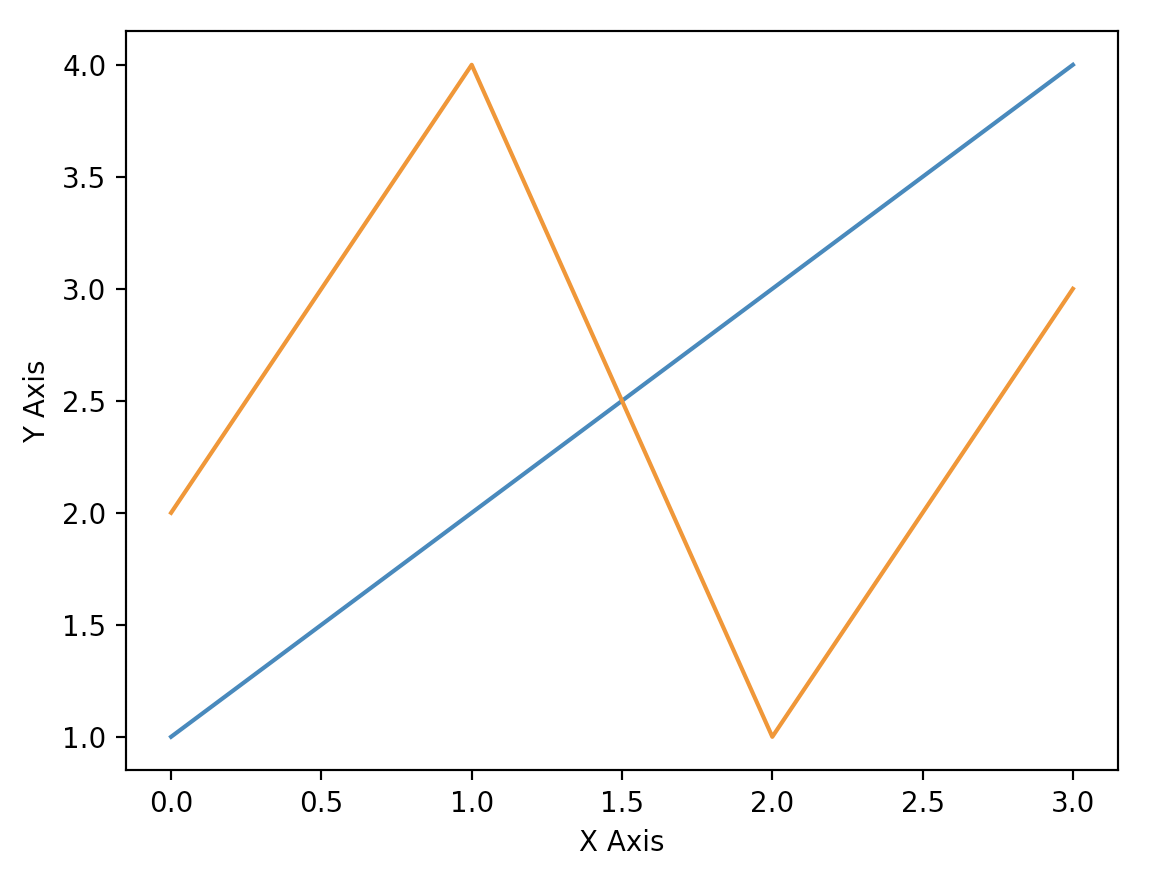You can change the color of the lines by adding color = ‘’ in the plt.plot function:
plt.plot([1, 2, 3, 4], color="green")

To add a title to your graph, you can call the function:
plt.title('Graph')

You can add a key to your graph by labeling the lines and then displaying what they mean in a legend like this:
import matplotlib.pyplot as plt

plt.title('Graph')

plt.plot([1, 2, 3, 4], label = 'Line 1') # The label informs people what the line
# represents
plt.plot([2, 4, 1, 3], label = 'Line 2')
plt.ylabel('Y Axis')
plt.xlabel('X Axis')

# The legend displays what the lines are
plt.legend(loc="lower left")

plt.show()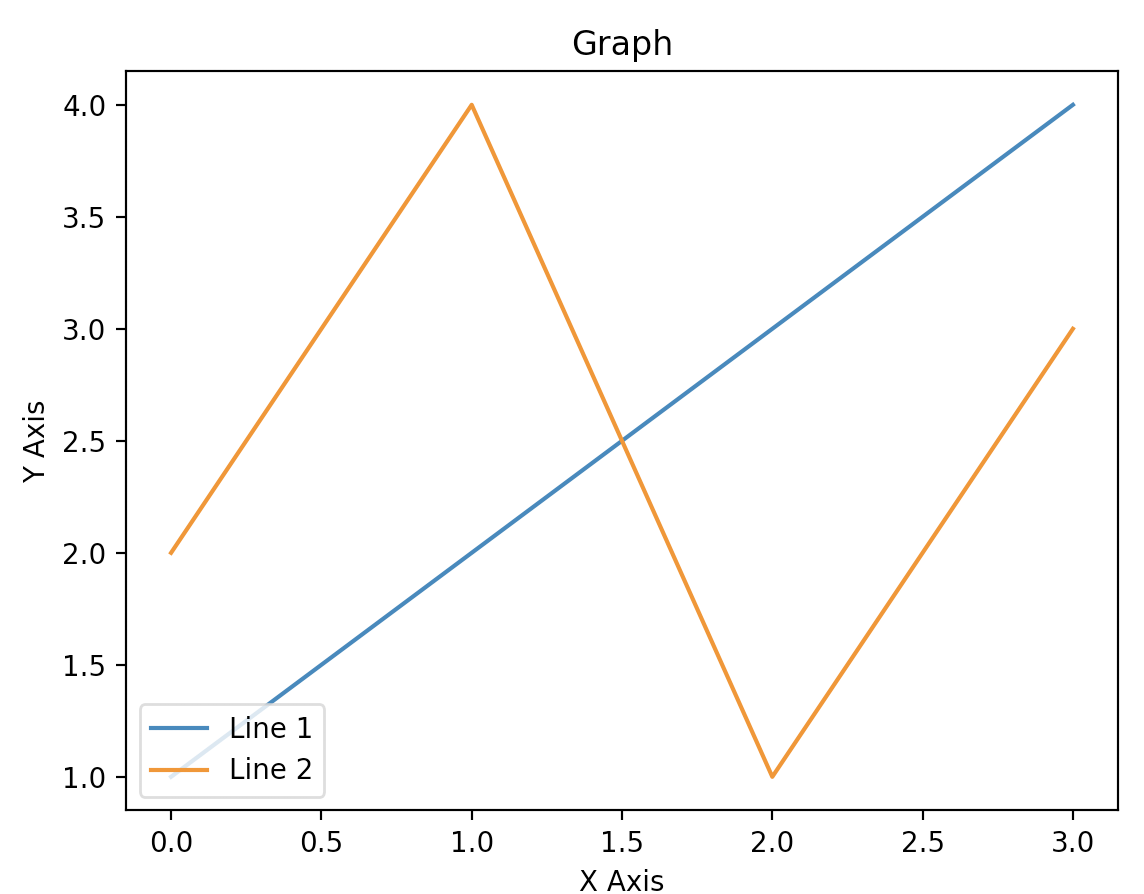Time
There is an extension package called time that allows you to wait for a certain amount of seconds before continuing on to the next function. To start using it write import time at the top of your code.
Then, to make it wait:
time.sleep(5) # The five represents how many seconds to wait
You can also put in a decimal if you would like to have your code wait less than a second. This package can be useful when you are trying to pint many things but want to give people a chance to read it all.
Pygame
Pygame is a python extension that allows you to create games in python! The first thing you need to do is install the extension by writing python3 -m pip install pygame in your computers terminal (not your python shell!)
Afterwards write pygame.init() which initializes all your imported modules.
Creating a Landscape
The next thing you should do is create a landscape in which you can code your game. You can do this by writing this code:
display_width = 800 # This is a variable that holds the width of our game landscape
display_hieght = 600 # This is a variable that holds the hieght of our game landscape

pygame.display.set_mode((display_width, display_hieght)) # This creates a space for us
# to make our game and sets the width and hieght to the variable we created earlier
This code would output this: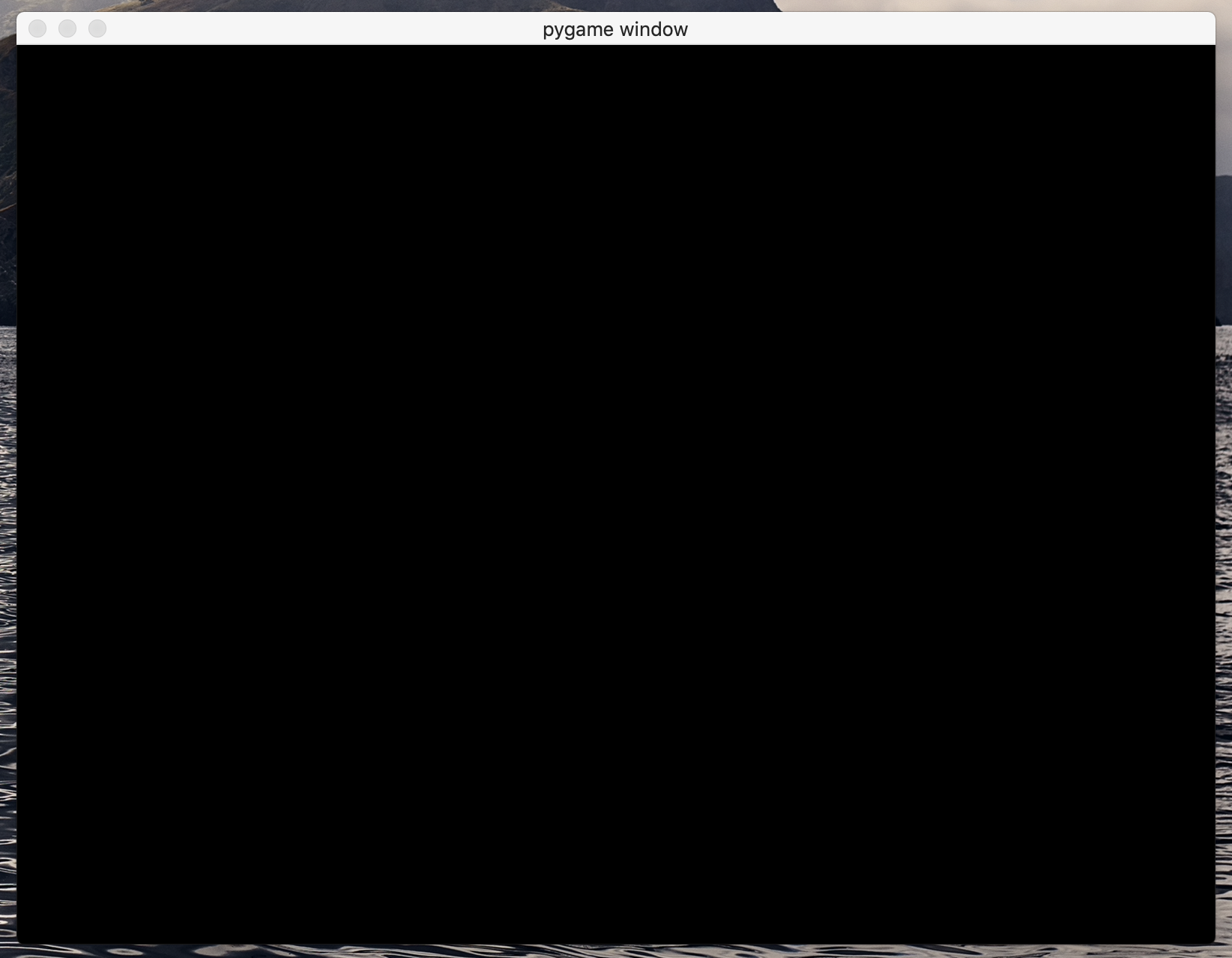Setting the Caption
The caption would replace the text “pygame window” with whatever you want to name your game. To set the caption, add this to your code:
pygame.display.set_caption("INSERT GAME NAME")
This would output: